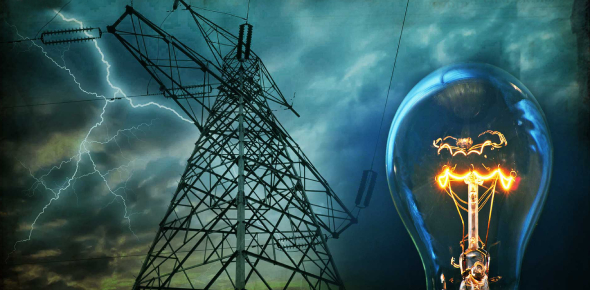# Interesting Questions On Electricity! Trivia Quiz

9 Questions | Total Attempts: 80SettingsLooking for some interesting questions on electricity? This quiz can show you the light. With this quiz, you will be responsible for knowing the material through which electricity flows easily, the most used voltage in the United States, how electrical power is measured in-branch units, and what a dimmer provides. This quiz will initiate a surge of knowledge regarding electricity. You can do it.

• 1.
The Flow of electricity is called______ and measured in_______
• A.

VOLTAGE

• B.

ELECTRICAL CURRENT

• C.

RESISTANCE

• D.

AMPS

• E.

VOLTS

• 2.
The material through which electricity flows easily
• A.

INSULATOR

• B.

CONDUCTOR

• C.

RESISTOR

• D.

COPPER

• E.

SWITCH

• 3.
The prevailing electrical current used in the U.S.
• A.

HERTZ

• B.

AC

• C.

DC

• D.

DIRECT CURRENT

• E.

ALTERNATING CURRENT

• 4.
Stronger than usual electrical current through the wires will create a ____________________ and potential for a _________________
• A.

PARALLEL CIRCUIT

• B.

SHORT CIRCUIT

• C.

FLOOD

• D.

SERIES CIRCUIT

• E.

FIRE

• 5.
The most common voltage used in the U.S. is ______________ with a cycle of  ___________ Hertz
• A.

50

• B.

Depending on the professional, the contractor or the owner involved in a project

• C.

60

• D.

115

• E.

120

• 6.
Electrical power/over time through branch circuits is measured in _____________ which is equal to
• A.

KILOWATT-HOURS

• B.

KILOWATT

• C.

100 Watts

• D.

120 Volts

• E.

1,000 Watts

• 7.
True or False: Comparing Life Cycle Cost: The greater initial cost usually amounts to a lower low life cycle cost.
• 8.
A dimmer provides variations in the intensity of an electrical light source by: 1. Varying the voltage  2. Varying the length of time that the current flows in an alternating cycle
• 9.
A dimmer provides variations in the intensity of an electrical light source by:
• A.

Allows current to flow at full voltage for a portion of the time

• B.

Varying the length of time that the current flows in an alternating cycle

• C.

Varying the voltage

• D.

Convert current into heat

• E.

Increases the life time of the lamp

Related TopicsBack to top